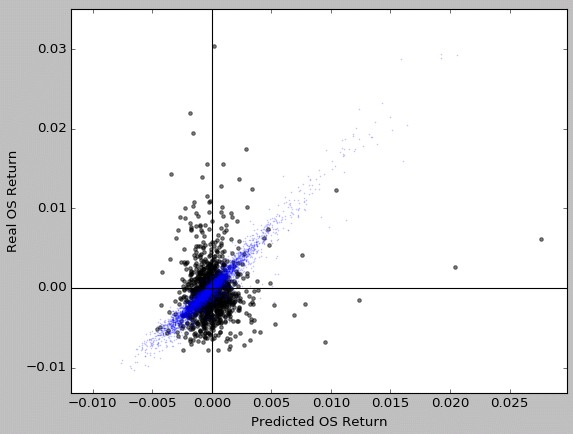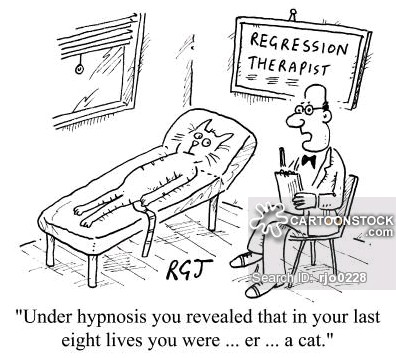## Looking at a Regressor for Out-of-sample predictions

Last week I talked about using a random classifier model for continuous out-of-sample predictions using the past three months of OS performance data to make a decision about whether a system is or is not likely to be profitable for the following three months. With the positive results we obtained with this approach in validation sets it is now interesting to ask about a regression approach and whether we could obtain results that are better than those we obtained when using a classifier. Since regressions can predict specific values if a regression approach achieves a low error it can be useful to decide exactly what set of systems to trade since for example only systems with high expected profitability could be chosen. In today’s article we are going to look at the results of a random forest regressor and whether it is in fact able to provide better results.I have never been successful at using regression algorithms to predict out-of-sample performance. The main reason why this is the case is mainly because there seems to be a large stochastic component in out-of-sample trading that causes attempts at very precise predictions to fail. If we try an unoptimized random forest regression approach using the same variables that were successful for classification we end up with the plot shown above. This is a classic example of over-fitting where the testing set – showed in blue – has very low errors while the validation set has very large errors. As you can see the scattering appears to be fundamentally random and there does not seem to be any significant accuracy when attempting to draw predictions for the next 3 months of out-of-sample trading. The mean absolute errors above are about 500-1500% of the values, meaning that if a mean return is predicted to be 1%, it could just as well be 11% or -10%.

However it is worth noting that the predictions are not randomly scattered. In fact if we consider the above regression based outputs and use them for classification (trade only predicted average returns > 0) we get results in the validation set that show positive improvements in the mean out-of-sample returns, positive but still lower than those that can be obtained with the normal optimized classification model. This hints that the regression model is able to capture some information even if the information it captures is not enough to yield predictions that don’t have the accuracy we would expect from a successful regression approach.We can also attempt to reduce over-fitting by reducing the maximum complexity of the random forest model to see if we can get better results. When we do this we obtain the plot showed above where – as you can see – the model is now not over fitting and the training and validation set results look fairly similar. This is in line with what we observed in the learning curves for our classifier where reducing complexity decreases the variance of the model but there isn’t a dramatic improvement in the results of the validation sets. The plot above also highlights why we can get better results from trading predictions than from simply trading the entire set, there is a significant improvement in the distribution of returns in the positive predictions compared to the general population available.

With some optimization the above regression approach can work almost as well as the optimized classifier on validation but its characteristics as a classifier are rather different with specificity and sensitivity values where the sensitivity is often higher than the specificity (usually 30% sensitivity with 20% specificity) while in the random forest classifier we are using right now these values – at the optimum probability threshold – are almost always inverted. The above means that the regression algorithm arrives at some useful predictions through a slightly different route which may increase the accuracy of our predictions if we use an ensemble. However – given that this regression approach completely fails as a regressor – it may be better to test ensembles using other classification algorithms such as gradient boosting.